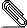# [NTG-context] \mframed not aligned in mkiv

Dear Hans,

I noticed a difference between mkii and mkiv in the behavior of \mframed: it
seems that in mkiv the frame is not vertically aligned with the the + sign in
the following example (or rather it is not vertically centered, please see the
attached PDF produced with ConTeXt  ver: 2013.10.15 13:52 MKIV).
Does one have to use now a new key to require this sort of alignment?

Best regard: OK

%%%% begin mframed-example.tex
\setupcolors[state=start]

\def\graymath{\mframed[frame=off,
background=color,
backgroundcolor=lightgray,
backgroundoffset=2pt
]}

\starttext

Since for $|x| < 1$ we have
\startformula
\log(1+x) = \graymath{x- \displaystyle{x^2\over2}} + {x^3 \over 3} + \cdots
\stopformula
we may write $\log(1+x) = x + O(x^2)$.

\stoptext
%%%% end mframed-example.texmframe.pdf
___________________________________________________________________________________
___________________________________________________________________________________